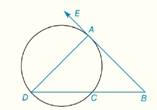Chapter 6.CR, Problem 10CRElementary Geometry For College St...

7th Edition
Alexander + 2 others
ISBN: 9781337614085

Solutions

Chapter
SectionElementary Geometry For College St...

7th Edition
Alexander + 2 others
ISBN: 9781337614085
Textbook Problem

In Review Exercises 5 to 10, B A → is tangent to the circle at point A in the figure shown. G i v e n :           m ∠ B = 35 °   a n d   m D C ^ = 70 °           F i n d :             m A D ^ ,   m A C ^To determine

To find: mAD^, mAC^

Explanation

Theorem:

1) The measure of an angle formed when two secants intersect at a point outside the circle is one-half the difference of the measures of two intercepted arcs.

2) The measure of an inscribed angle of a circle is one-half the measure of its intercepted arc.

Calculation:

Given that

mB=35°, mDC^=70° and BA is tangent to the circle at point A.

By theorem, the measure of an angle (mB) formed when two secants (BD and BA) intersect at a point (B) outside the circle is one-half the difference of the measures of two intercepted arcs AD^ and AC^ .

Still sussing out bartleby?

Check out a sample textbook solution.

See a sample solution

The Solution to Your Study Problems

Bartleby provides explanations to thousands of textbook problems written by our experts, many with advanced degrees!

Get Started

Perform each operation and simplify: a. (x2+1)(12x1/2)x1/2(2x)(x2+1)2 b. 3xx+2+3x+2

Applied Calculus for the Managerial, Life, and Social Sciences: A Brief Approach

Evaluate the integral. 33x1+|x|dx

Calculus (MindTap Course List)

Prove that limx0+lnx=

Calculus: Early Transcendentals

Evaluate the indefinite integral. 1+x1+x2dx

Single Variable Calculus: Early Transcendentals